# Modelling Ocean surface waves

Trying to model Ocean surface waves, I have played using a linear superposition of sinusoids and also on the fact that phase speed (following a wave's crest) is twice as fast as group speed (following a group of waves). Finally, I more recently used such generation of a model of the random superposition of waves to model the wigggling lines formed by the refraction (bendings of the trajectory of a ray at the interface between air and water) of light, called caustics...

Observing real-life ocean waves instructed me that if a single wave is well approximated by a sinusoïd, each wave is qualitatively a bit different. Typical for these surface gravity waves are the sharp crests and flat troughs. As a matter of fact, modelling ocean waves is on one side very useful (Ocean dynamics and its impact on climate, modelling tides, tsunamis, diffraction in a bay to predict coastline evolution, ...) but quite demanding despite a well known mathematical model. Starting with the Navier-Stokes equations to an incompressible fluid (water) in a gravitational field leads to Luke's variational principle in certain simplifying conditions. Further simplifications lead to the approximate solution given by Stokes which gives the following shape as the sum of different harmonics:This seems well enough at the moment and I will capture this shape in this notebook and notably if that applies to a random mixture of such waves...

(WORK IN PROGRESS)

The current situation is that these solutions seem to not fit what is displayed on the wikipedia page and that I do not spot the bug I may have introduced... More work on this is needed and any feedback is welcome...

Let's first initialize the notebook:

In :
import numpy as np
np.set_printoptions(precision=6, suppress=True)
import matplotlib.pyplot as plt
phi = (np.sqrt(5)+1)/2
fig_width = 10
figsize = (fig_width, fig_width/phi)


### From a sinusoid to a Stoke wave¶

From the equation given for third-order Stokes wave on deep water, we can write the following function:

In :
def stokes(pos, a=.3/2/np.pi, k=2*np.pi):
# k*a is a dimensionless expansion parameter
elevation = (1-1/16*(k*a)**2) * np.cos(k*pos)
elevation += 1/2*(k*a)**1 * np.cos(2*k*pos)
elevation += 3/8*(k*a)**2 * np.cos(3*k*pos)
return a * elevation


Let's define the position along one period and one sample wave with wave steepness $k \cdot a = 0.3$:

In :
N_pos = 501
pos = np.linspace(0, 1, N_pos, endpoint=True)
fig, ax = plt.subplots(figsize=(fig_width, fig_width/phi**3))
ax.plot(pos, stokes(pos))
ax.set_xlim(np.min(pos), np.max(pos));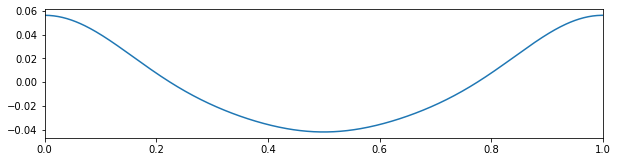We can observe the effect of wave height from sinusoid (small $a$) to a Stokes wave (higher $a$):

In :
fig, ax = plt.subplots(figsize=(fig_width, fig_width/phi**3))
for a in np.linspace(.001, .2, 10, endpoint=True):
elevation = stokes(pos, a=a)
ax.plot(pos, elevation)
ax.set_xlim(np.min(pos), np.max(pos));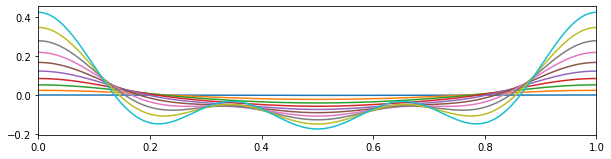Normalized, we get:

In :
fig, ax = plt.subplots(figsize=(fig_width, fig_width/phi**3))
for a in np.linspace(.001, .2, 10, endpoint=True):
elevation = stokes(pos, a=a)
ax.plot(pos, elevation/np.max(np.abs(elevation)))
ax.set_xlim(np.min(pos), np.max(pos));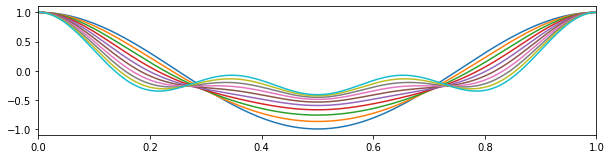Which looks nice but also shows that the approximation is not valid for higher amplitudes:

In :
fig, ax = plt.subplots(figsize=(fig_width, fig_width/phi**3))
for a in np.logspace(-3, 0, 10, base=10, endpoint=True):
elevation = stokes(pos, a=a)
ax.plot(pos, elevation/np.max(np.abs(elevation)))
ax.set_xlim(np.min(pos), np.max(pos));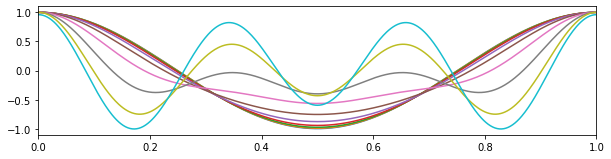### Fifth order Fenton wave¶

Following the 5th order soltuion, we can write the following function with expansion parameter $\epsilon = k\cdot H / 2$ (Eq. 18):

In :
def fenton(pos, H=.141*2/2/np.pi, k=2*np.pi):
eps = k * H / 2 # dimensionless expansion parameter
elevation = eps * np.cos(k*pos)
elevation += 1/2 * eps**2 * np.cos(2*k*pos)
elevation += 3/8 * eps**3 * (np.cos(3*k*pos)-np.cos(k*pos))
elevation += 1/3 * eps**4 * (np.cos(2*k*pos)+np.cos(4*k*pos))
elevation += 1/384 * eps**5 * (-422*np.cos(k*pos) + 297 * np.cos(3*k*pos)+125*np.cos(5*k*pos))
return elevation / k


Note that if we keep the three first orders, this can also be written with $H = 2 \cdot a \cdot ( 1 + \frac 3 8 \cdot k^2 \cdot a^2 )$ and thus $\epsilon = ( k\cdot a ) \cdot ( 1 + \frac 3 8 \cdot (k \cdot a)^2 )$:

In :
def fenton_approx_1(pos, a, k):
elevation = ((k*a) + 3 / 8 * (k*a)**3) * np.cos(k*pos)
elevation += 1/2 * ((k*a) + 3 / 8 * (k*a)**3)**2 * np.cos(2*k*pos)
elevation += 3/8 * ((k*a) + 3 / 8 * (k*a)**3)**3 * (np.cos(3*k*pos)-np.cos(k*pos))
return elevation / k


keeping only terms with orders less than $3$, we obtain :

In :
def fenton_approx_2(pos, a, k):
elevation = (k*a) * np.cos(k*pos)
elevation += 1/2 * (k*a)**2 * np.cos(2*k*pos)
elevation += 3/8 * (k*a)**3 * (np.cos(3*k*pos)-np.cos(k*pos)) + 3 / 8 * (k*a)**3 * np.cos(k*pos)
return elevation / k


By the way, this is consistant with the third order Stokes approximation defined above as:

In :
def stokes(pos, a, k):
elevation = (1-1/16*(k*a)**2) * np.cos(k*pos)
elevation += 1/2*(k*a) * np.cos(2*k*pos)
elevation += 3/8*(k*a)**2 * np.cos(3*k*pos)
return a * elevation


Let's define the position along one period and one sample wave with wave steepness $\epsilon = 0.141$:

In :
fig, ax = plt.subplots(figsize=(fig_width, fig_width/phi**3))
ax.plot(pos, fenton(pos))
ax.set_xlim(np.min(pos), np.max(pos));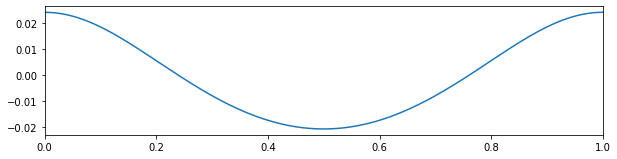In :
fig, ax = plt.subplots(figsize=(fig_width, fig_width/phi**3))
for H in np.logspace(-3, -.58, 10, base=10, endpoint=True):
elevation = fenton(pos, H=H)
ax.plot(pos, elevation/np.max(np.abs(elevation)))
ax.set_xlim(np.min(pos), np.max(pos));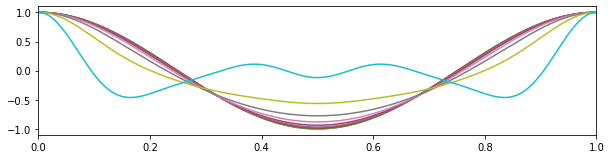### parametrized ocean spectrum¶

Another solution would be to transform the wave using the formulation of speed: https://people.cs.clemson.edu/~jtessen/reports/papers_files/course_slides2002.pdf https://www.math.arizona.edu/~zakharov/3Articles/3ZakKuz97.pdf

Let's define ocean's spectrum following that matlab code:

In [ ]:
A = 1e+4
g = 9.81 # gravitational constant
windSpeed = 1e+2
def phillips(kx, ky, windDir, windSpeed, A, g):
k_sq = kx^2 + ky^2;
L = windSpeed^2 / g;
k = [kx, ky] / sqrt(k_sq);
wk = k(1) * windDir(1) + k(2) * windDir(2)
P = A / k_sq^2 * exp(-1.0 / (k_sq * L^2)) * wk^2
return P


### some book keeping for the notebook¶

In :
%load_ext watermark
%watermark -i -h -m -v -p numpy,matplotlib,scipy,pillow,imageio  -r -g -b

Python implementation: CPython
Python version       : 3.9.7
IPython version      : 7.27.0

numpy     : 1.20.3
matplotlib: 3.3.3
scipy     : 1.6.0
pillow    : not installed
imageio   : 2.9.0

Compiler    : Clang 12.0.5 (clang-1205.0.22.9)
OS          : Darwin
Release     : 20.6.0
Machine     : x86_64
Processor   : i386
CPU cores   : 4
Architecture: 64bit

Hostname: ekla

Git hash: d75299798ff8970f1d314d97d85bd22dc496df4f

Git repo: https://github.com/laurentperrinet/sciblog.git

Git branch: master